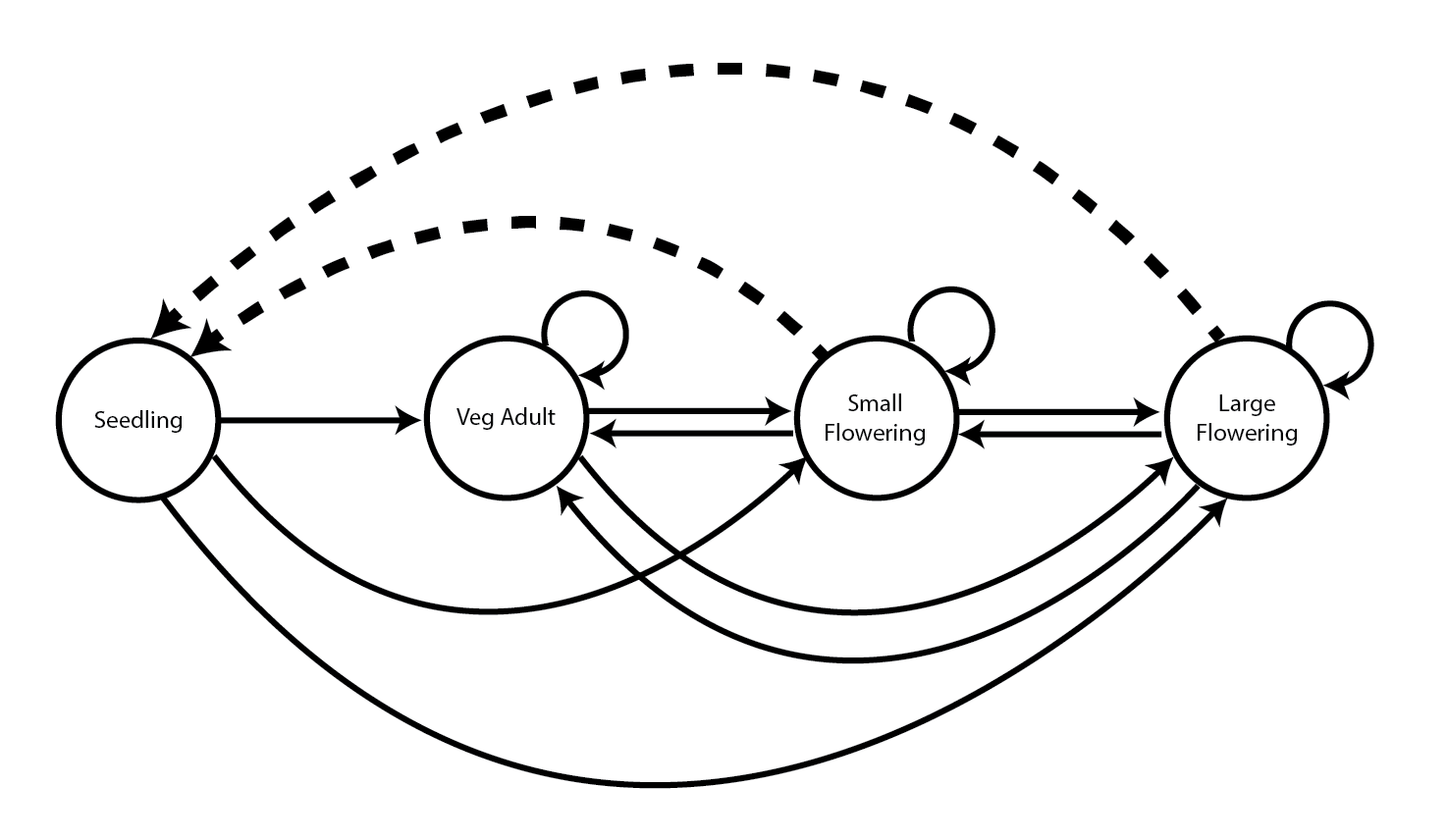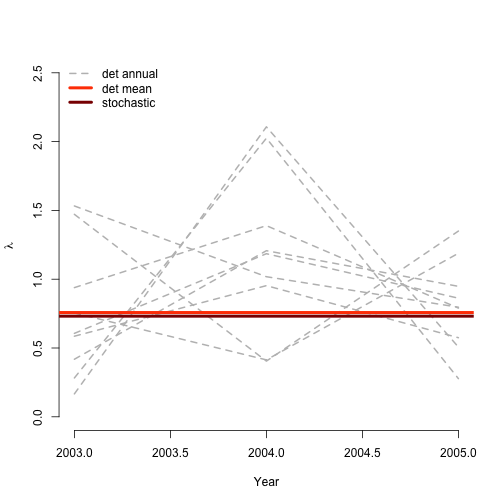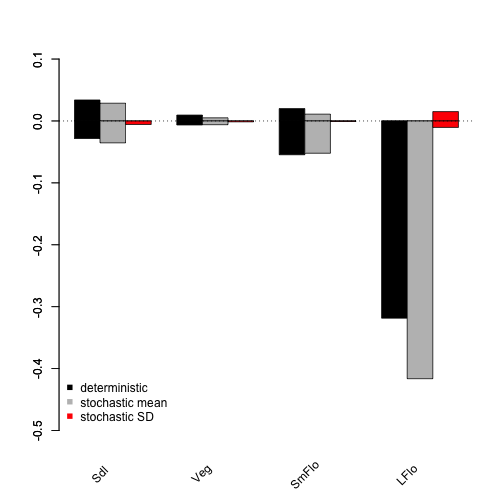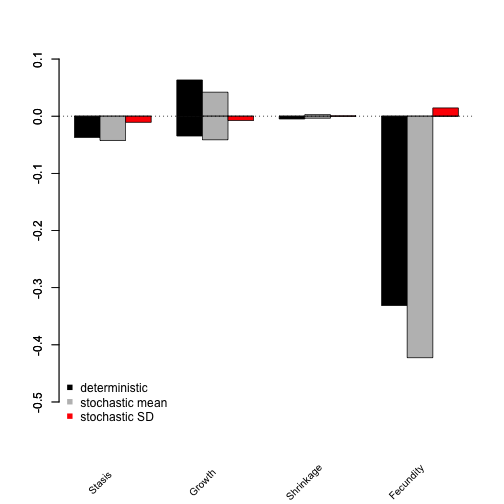# LTREs, sLTREs, and MPM import with Anthyllis vulneraria

In this vignette, we will import a MPM created elsewhere into lefko3 and analyze it via LTRE and sLTRE analysis. These are inherently ahistorical MPMs, and so we do not include historical analyses in this vignette. To reduce output size, we have prevented some statements from running if they produce long stretches of output. Examples include most summary() calls. In these cases, we include hashtagged versions of these calls, and our text assumes that the user runs these statements without hashtags. This vignette is only a sample analysis. Detailed information and instructions on using lefko3 are available through a free online e-book called lefko3: a gentle introduction, available on the projects page of the Shefferson lab website. This website also includes links to long-format vignettes and other demonstrations of this package.

### ORGANISM AND POPULATIONS

Davison et al. (2010) reported stochastic contributions made by differences in vital rate means and variances among nine natural populations of the perennial herb Anthyllis vulneraria, also known as kidney vetch, occurring in calcareous grasslands in the Viroin Valley of southwestern Belgium. A. vulneraria is a grassland specialist and the unique host plant of the Red-listed blue butterfly (Cupido minimus). It is a short-lived, rosette-forming legume with a complex life cycle including stasis and retrogression between four stages but no seedbank (seedlings, juveniles, small adults and large adults; Figure 9.1).Figure 9.1. Life history model of Anthyllis vulneraria. Solid arrows indicate survival transitions while dashed arrows indicate fecundity transitions.

Nine populations (N = 27-50,000) growing in distinct grassland fragments were surveyed between 2003 and 2006, yielding three (4x4) annual transition matrices for each population. The populations occurred within grassland fragments, and were mostly managed as nature reserves through rotational sheep grazing. These surveys coincided with a summer heat wave (2003), followed by a spring drought (2005) and an even more extreme heat wave (2006). These populations have been subject to detailed study for aspects of their genetics and demography, and further details on the sites can be obtained through the resulting publications (Krauss, Steffan-Dewenter, and Tscharntke 2004; Honnay et al. 2006; Piessens et al. 2009).

### BASIC WORKFLOW

Our goal in this exercise will be to import the published MPMs available for these nine populations of Anthyllis vulneraria, and to analyze the demographic differences between populations using deterministic and stochastic life table response experiments (LTRE and sLTRE). We will use the matrices analyzed in Davison et al. (2010), and attempt to reproduce their results.

#### Step 1. Life history model development

We will first need to describe the life history characterizing the dataset, matching it to our analyses properly with a stageframe. Since we do not have the original demographic dataset that produced the published matrices, we do not need to know the exact sizes of plants and so will use proxy values. These proxy values need to be unique and non-negative, and they need non-overlapping bins usable as size classes defining each stage. However, since we are not analyzing size itself here, they do not need any further basis in reality. Other characteristics must be exact and realistic to make sure that the analyses work properly, including all other stage descriptions such as reproductive status, propagule status, and observation status.

sizevector <- c(1, 1, 2, 3) # These sizes are not from the original paper
stagevector <- c("Sdl", "Veg", "SmFlo", "LFlo")
repvector <- c(0, 0, 1, 1)
obsvector <- c(1, 1, 1, 1)
matvector <- c(0, 1, 1, 1)
immvector <- c(1, 0, 0, 0)
propvector <- c(0, 0, 0, 0)
indataset <- c(1, 1, 1, 1)
binvec <- c(0.5, 0.5, 0.5, 0.5)
"Large flowering")

anthframe <- sf_create(sizes = sizevector, stagenames = stagevector,
repstatus = repvector, obsstatus = obsvector, matstatus = matvector,
immstatus = immvector, indataset = indataset, binhalfwidth = binvec,
#anthframe

#### Step 2. Data organization

Next we will enter the data for this vignette. Our data is in the form of matrices published in Davison et al. (2010). We will enter these matrices as standard R matrix class objects. All matrices are square with 4 columns, and we fill them by row. As an example, let’s load the first matrix and take a look at it.

XC3 <- matrix(c(0, 0, 1.74, 1.74,     # POPN C 2003-2004
0.208333333, 0, 0, 0.057142857,
0.041666667, 0.076923077, 0, 0,
0.083333333, 0.076923077, 0.066666667, 0.028571429), 4, 4, byrow = TRUE)
XC3
#>            [,1]       [,2]       [,3]       [,4]
#> [1,] 0.00000000 0.00000000 1.74000000 1.74000000
#> [2,] 0.20833333 0.00000000 0.00000000 0.05714286
#> [3,] 0.04166667 0.07692308 0.00000000 0.00000000
#> [4,] 0.08333333 0.07692308 0.06666667 0.02857143

These are A matrices, meaning that they include all survival-transitions and fecundity for the population as a whole. The corresponding U and F matrices were not provided in that paper, although it is most likely that the elements valued at 1.74 in the top right-hand corner are only composed of fecundity values while the rest of the matrix is only composed of survival transitions (this might not be the case if clonal reproduction were possible). The order of rows and columns corresponds to the order of stages in the stageframe anthframe. Let’s now load the remaining matrices.

XC4 <- matrix(c(0, 0, 0.3, 0.6,     # POPN C 2004-2005
0.32183908, 0.142857143, 0, 0,
0.16091954, 0.285714286, 0, 0,
0.252873563, 0.285714286, 0.5, 0.6), 4, 4, byrow = TRUE)

XC5 <- matrix(c(0, 0, 0.50625, 0.675,     # POPN C 2005-2006
0, 0, 0, 0.035714286,
0.1, 0.068965517, 0.0625, 0.107142857,
0.3, 0.137931034, 0, 0.071428571), 4, 4, byrow = TRUE)

XE3 <- matrix(c(0, 0, 2.44, 6.569230769,     # POPN E 2003-2004
0.196428571, 0, 0, 0,
0.125, 0.5, 0, 0,
0.160714286, 0.5, 0.133333333, 0.076923077), 4, 4, byrow = TRUE)

XE4 <- matrix(c(0, 0, 0.45, 0.646153846,     # POPN E 2004-2005
0.06557377, 0.090909091, 0.125, 0,
0.032786885, 0, 0.125, 0.076923077,
0.049180328, 0, 0.125, 0.230769231), 4, 4, byrow = TRUE)

XE5 <- matrix(c(0, 0, 2.85, 3.99,     # POPN E 2005-2006
0.083333333, 0, 0, 0,
0, 0, 0, 0,
0.416666667, 0.1, 0, 0.1), 4, 4, byrow = TRUE)

XF3 <- matrix(c(0, 0, 1.815, 7.058333333,     # POPN F 2003-2004
0.075949367, 0, 0.05, 0.083333333,
0.139240506, 0, 0, 0.25,
0.075949367, 0, 0, 0.083333333), 4, 4, byrow = TRUE)

XF4 <- matrix(c(0, 0, 1.233333333, 7.4,     # POPN F 2004-2005
0.223880597, 0, 0.111111111, 0.142857143,
0.134328358, 0.272727273, 0.166666667, 0.142857143,
0.119402985, 0.363636364, 0.055555556, 0.142857143), 4, 4, byrow = TRUE)

XF5 <- matrix(c(0, 0, 1.06, 3.372727273,     # POPN F 2005-2006
0.073170732, 0.025, 0.033333333, 0,
0.036585366, 0.15, 0.1, 0.136363636,
0.06097561, 0.225, 0.166666667, 0.272727273), 4, 4, byrow = TRUE)

XG3 <- matrix(c(0, 0, 0.245454545, 2.1,     # POPN G 2003-2004
0, 0, 0.045454545, 0,
0.125, 0, 0.090909091, 0,
0.125, 0, 0.090909091, 0.333333333), 4, 4, byrow = TRUE)

XG4 <- matrix(c(0, 0, 1.1, 1.54,     # POPN G 2004-2005
0.111111111, 0, 0, 0,
0, 0, 0, 0,
0.111111111, 0, 0, 0), 4, 4, byrow = TRUE)

XG5 <- matrix(c(0, 0, 0, 1.5,     # POPN G 2005-2006
0, 0, 0, 0,
0.090909091, 0, 0, 0,
0.545454545, 0.5, 0, 0.5), 4, 4, byrow = TRUE)

XL3 <- matrix(c(0, 0, 1.785365854, 1.856521739,     # POPN L 2003-2004
0.128571429, 0, 0, 0.010869565,
0.028571429, 0, 0, 0,
0.014285714, 0, 0, 0.02173913), 4, 4, byrow = TRUE)

XL4 <- matrix(c(0, 0, 14.25, 16.625,     # POPN L 2004-2005
0.131443299, 0.057142857, 0, 0.25,
0.144329897, 0, 0, 0,
0.092783505, 0.2, 0, 0.25), 4, 4, byrow = TRUE)

XL5 <- matrix(c(0, 0, 0.594642857, 1.765909091,     # POPN L 2005-2006
0, 0, 0.017857143, 0,
0.021052632, 0.018518519, 0.035714286, 0.045454545,
0.021052632, 0.018518519, 0.035714286, 0.068181818), 4, 4, byrow = TRUE)

XO3 <- matrix(c(0, 0, 11.5, 2.775862069,     # POPN O 2003-2004
0.6, 0.285714286, 0.333333333, 0.24137931,
0.04, 0.142857143, 0, 0,
0.16, 0.285714286, 0, 0.172413793), 4, 4, byrow = TRUE)

XO4 <- matrix(c(0, 0, 3.78, 1.225,     # POPN O 2004-2005
0.28358209, 0.171052632, 0, 0.166666667,
0.084577114, 0.026315789, 0, 0.055555556,
0.139303483, 0.447368421, 0, 0.305555556), 4, 4, byrow = TRUE)

XO5 <- matrix(c(0, 0, 1.542857143, 1.035616438,     # POPN O 2005-2006
0.126984127, 0.105263158, 0.047619048, 0.054794521,
0.095238095, 0.157894737, 0.19047619, 0.082191781,
0.111111111, 0.223684211, 0, 0.356164384), 4, 4, byrow = TRUE)

XQ3 <- matrix(c(0, 0, 0.15, 0.175,     # POPN Q 2003-2004
0, 0, 0, 0,
0, 0, 0, 0,
1, 0, 0, 0), 4, 4, byrow = TRUE)

XQ4 <- matrix(c(0, 0, 0, 0.25,     # POPN Q 2004-2005
0, 0, 0, 0,
0, 0, 0, 0,
1, 0.666666667, 0, 1), 4, 4, byrow = TRUE)

XQ5 <- matrix(c(0, 0, 0, 1.428571429,     # POPN Q 2005-2006
0, 0, 0, 0.142857143,
0.25, 0, 0, 0,
0.25, 0, 0, 0.571428571), 4, 4, byrow = TRUE)

XR3 <- matrix(c(0, 0, 0.7, 0.6125,     # POPN R 2003-2004
0.25, 0, 0, 0.125,
0, 0, 0, 0,
0.25, 0.166666667, 0, 0.25), 4, 4, byrow = TRUE)

XR4 <- matrix(c(0, 0, 0, 0.6,     # POPN R 2004-2005
0.285714286, 0, 0, 0,
0.285714286, 0.333333333, 0, 0,
0.285714286, 0.333333333, 0, 1), 4, 4, byrow = TRUE)

XR5 <- matrix(c(0, 0, 0.7, 0.6125,     # POPN R 2005-2006
0, 0, 0, 0,
0, 0, 0, 0,
0.333333333, 0, 0.333333333, 0.625), 4, 4, byrow = TRUE)

XS3 <- matrix(c(0, 0, 2.1, 0.816666667,     # POPN S 2003-2004
0.166666667, 0, 0, 0,
0, 0, 0, 0,
0, 0, 0, 0.166666667), 4, 4, byrow = TRUE)

XS4 <- matrix(c(0, 0, 0, 7,     # POPN S 2004-2005
0.333333333, 0.5, 0, 0,
0, 0, 0, 0,
0.333333333, 0, 0, 1), 4, 4, byrow = TRUE)

XS5 <- matrix(c(0, 0, 0, 1.4,     # POPN S 2005-2006
0, 0, 0, 0,
0, 0, 0, 0.2,
0.111111111, 0.75, 0, 0.2), 4, 4, byrow = TRUE)

We will incorporate all of these matrices into a lefkoMat object. To do so, we will first organize the matrices in the proper order within a list. Then, we will create the lefkoMat object to hold these matrices by calling function create_lM() with the list object we created. We will also include metadata describing the order of populations (here treated as patches), and the order of monitoring occasions.

mats_list <- list(XC3, XC4, XC5, XE3, XE4, XE5, XF3, XF4, XF5, XG3, XG4, XG5,
XL3, XL4, XL5, XO3, XO4, XO5, XQ3, XQ4, XQ5, XR3, XR4, XR5, XS3, XS4, XS5)

anth_lefkoMat <- create_lM(mats_list, anthframe, hstages = NA,
historical = FALSE, poporder = 1,
patchorder = c(1, 1, 1, 2, 2, 2, 3, 3, 3, 4, 4, 4, 5, 5, 5, 6, 6, 6, 7, 7, 7,
8, 8, 8, 9, 9, 9),
yearorder = c(2003, 2004, 2005, 2003, 2004, 2005, 2003, 2004, 2005, 2003,
2004, 2005, 2003, 2004, 2005, 2003, 2004, 2005, 2003, 2004, 2005, 2003,
2004, 2005, 2003, 2004, 2005))
#anth_lefkoMat

The resulting object has all of the elements of a standard lefkoMat object except for those elements related to quality control in the demographic dataset and linear modeling. The option UFdecomp was left at its default (UFdecomp = TRUE), and so create_lM() used the stageframe to infer where fecundity values were located in the matrices and created U and F matrices separating those values. The default option for historical is set to FALSE, yielding an NA in place of the hstages element, which would typically list the order of historical stage pairs.

#summary(anth_lefkoMat)

The summary of this new lefkoMat object shows us that we have 27 matrices with 4 rows and columns each. The estimated numbers of transitions corresponds to the non-zero entries in each matrix. We see that we are covering 1 population, 9 patches, and 3 time steps. All of the survival probabilities observed fall within the bounds of 0 to 1, so everything seems alright.

#### Step 3. MPM analysis

Now, we will develop arithmetic mean matrices and assess the deterministic and stochastic population growth rates, $$\lambda$$ and $$a$$.

anth_lmean <- lmean(anth_lefkoMat)

lambda2 <- lambda3(anth_lefkoMat)
lambda2m <- lambda3(anth_lmean)
set.seed(42)
sl2 <- slambda3(anth_lefkoMat) #Stochastic growth rate
sl2$expa <- exp(sl2$a)

plot(lambda ~ year2, data = subset(lambda2, patch == 1), ylim = c(0, 2.5),xlab = "Year",
ylab = expression(lambda), type = "l", col = "gray", lty= 2, lwd = 2, bty = "n")
lines(lambda ~ year2, data = subset(lambda2, patch == 2), col = "gray", lty= 2, lwd = 2)
lines(lambda ~ year2, data = subset(lambda2, patch == 3), col = "gray", lty= 2, lwd = 2)
lines(lambda ~ year2, data = subset(lambda2, patch == 4), col = "gray", lty= 2, lwd = 2)
lines(lambda ~ year2, data = subset(lambda2, patch == 5), col = "gray", lty= 2, lwd = 2)
lines(lambda ~ year2, data = subset(lambda2, patch == 6), col = "gray", lty= 2, lwd = 2)
lines(lambda ~ year2, data = subset(lambda2, patch == 7), col = "gray", lty= 2, lwd = 2)
lines(lambda ~ year2, data = subset(lambda2, patch == 8), col = "gray", lty= 2, lwd = 2)
lines(lambda ~ year2, data = subset(lambda2, patch == 9), col = "gray", lty= 2, lwd = 2)
abline(a = lambda2m$lambda, b = 0, lty = 1, lwd = 4, col = "orangered") abline(a = sl2$expa, b = 0, lty = 1, lwd = 4, col = "darkred")
legend("topleft", c("det annual", "det mean", "stochastic"), lty = c(2, 1, 1),
col = c("gray", "orangered", "darkred"), lwd = c(2, 4, 4), bty = "n")Figure 9.2. Deterministic vs. stochastic lambda

Clearly, these populations exhibit extremely variable growth across the short field study during which they were monitored. Also very clear is that these populations appear to be on the decline, as shown by $$\lambda$$ for the overall mean matrix, and $$e$$ to the power of the stochastic growth rate $$a = \text{log} \lambda$$.

Let’s conduct a life table response experiment (LTRE) next. This will be a deterministic LTRE, meaning that we will assess the impacts of differences in matrix elements between the core matrices input via the lefkoMat object anth_lefkoMat and the overall arithmetic grand mean matrix on differences in the deterministic growth rate, $$\lambda$$. We could use a different reference matrix if we wished, but the default is to use the arithmetic grand mean. Here, because we cannot analyze temporal shifts using a deterministic LTRE, we will utilize the mean matrix set instead.

trialltre_det <- ltre3(anth_lmean, stochastic = FALSE, steps = 10000,
time_weights = NA, sparse = "auto")
#> Warning: Matrices input as mats will also be used as reference matrices.
#> Using all refmats matrices as reference matrices.
#trialltre_det

The resulting lefkoLTRE object gives the LTRE contributions for each matrix relative to the arithmetic grand mean matrix. While the differences in LTRE contributions across space are of interest, we cannot really infer differences across time this way because, across time, matrices are not related additively. To assess the contributions across space while accounting for temporal shifts, we should conduct a stochastic LTRE (sLTRE) on the original annual matrices, as below.

trialltre_sto <- ltre3(anth_lefkoMat, stochastic = TRUE, steps = 10000,
time_weights = NA, sparse = "auto")
#> Warning: Matrices input as mats will also be used as reference matrices.
#> Using all refmats matrices as reference matrices.
#trialltre_sto

The sLTRE produces output that is a bit different from the deterministic LTRE output. In the output, we see two lists of matrices prior to the MPM metadata. The first, ltre_mean, is a list of matrices showing the impact of differences in mean elements between the patch-level temporal mean matrices and the reference temporal mean matrix. The second, ltre_sd, is a list of matrices showing the impact of differences in the temporal standard deviation of each element between the patch-level and reference matrix sets. In other words, while a standard LTRE shows the impact of changes in matrix elements on $$\lambda$$, the sLTRE shows the impacts of changes in the temporal mean and variability of matrix elements on $$\text{log} \lambda$$. The labels element shows the order of matrices with reference to the populations or patches (remember that here, the populations are referred to as patches).

The output objects are large and can take a great deal of effort to look over and understand. Therefore, we will show three approaches to assessing these objects, using an approach similar to that used to assess elasticities. These methods can be used to assess patterns in all 9 populations, but for brevity we will focus only on the first population here. First, we will identify the elements most strongly impacting the population growth rate in each case.

writeLines(paste0("Highest (i.e most positive) deterministic LTRE contribution: ",
max(trialltre_det$ltre_det[]))) #> Highest (i.e most positive) deterministic LTRE contribution: 0.020993880824138 writeLines(paste0("Highest deterministic LTRE contribution is associated with element: ", which(trialltre_det$ltre_det[] == max(trialltre_det$ltre_det[])))) #> Highest deterministic LTRE contribution is associated with element: 3 writeLines(paste0("Lowest (i.e. most negative) deterministic LTRE contribution: ", min(trialltre_det$ltre_det[])))
#> Lowest (i.e. most negative) deterministic LTRE contribution: -0.278604545412243
writeLines(paste0("Lowest deterministic LTRE contribution is associated with element: ",
which(trialltre_det$ltre_det[] == min(trialltre_det$ltre_det[]))))
#> Lowest deterministic LTRE contribution is associated with element: 13

writeLines(paste0("Highest stochastic mean LTRE contribution: ",
max(trialltre_sto$ltre_mean[]))) #> Highest stochastic mean LTRE contribution: 0.0179472041741181 writeLines(paste0("Highest stochastic mean LTRE contribution is associated with element: ", which(trialltre_sto$ltre_mean[] == max(trialltre_sto$ltre_mean[])))) #> Highest stochastic mean LTRE contribution is associated with element: 3 writeLines(paste0("Lowest stochastic mean LTRE contribution: ", min(trialltre_sto$ltre_mean[])))
#> Lowest stochastic mean LTRE contribution: -0.370937926897558
writeLines(paste0("Lowest stochastic mean LTRE contribution is associated with element: ",
which(trialltre_sto$ltre_mean[] == min(trialltre_sto$ltre_mean[]))))
#> Lowest stochastic mean LTRE contribution is associated with element: 13

writeLines(paste0("Highest stochastic SD LTRE contribution: ",
max(trialltre_sto$ltre_sd[]))) #> Highest stochastic SD LTRE contribution: 0.014441782311314 writeLines(paste0("Highest stochastic SD LTRE contribution is associated with element: ", which(trialltre_sto$ltre_sd[] == max(trialltre_sto$ltre_sd[])))) #> Highest stochastic SD LTRE contribution is associated with element: 13 writeLines(paste0("Lowest stochastic SD LTRE contribution: ", min(trialltre_sto$ltre_sd[])))
#> Lowest stochastic SD LTRE contribution: -0.0103367392514045
writeLines(paste0("Lowest stochastic SD LTRE contribution is associated with element: ",
which(trialltre_sto$ltre_sd[] == min(trialltre_sto$ltre_sd[]))))
#> Lowest stochastic SD LTRE contribution is associated with element: 16

writeLines(paste0("Total positive deterministic LTRE contributions: ",
sum(trialltre_det$ltre_det[][which(trialltre_det$ltre_det[] > 0)])))
#> Total positive deterministic LTRE contributions: 0.0633856585971784
writeLines(paste0("Total negative deterministic LTRE contributions: ",
sum(trialltre_det$ltre_det[][which(trialltre_det$ltre_det[] < 0)])))
#> Total negative deterministic LTRE contributions: -0.407957287954865
writeLines(paste0("Total positive stochastic mean LTRE contributions: ",
sum(trialltre_sto$ltre_mean[][which(trialltre_sto$ltre_mean[] > 0)])))
#> Total positive stochastic mean LTRE contributions: 0.0447245785886143
writeLines(paste0("Total negative stochastic mean LTRE contributions: ",
sum(trialltre_sto$ltre_mean[][which(trialltre_sto$ltre_mean[] < 0)])))
#> Total negative stochastic mean LTRE contributions: -0.509757776771075
writeLines(paste0("Total positive stochastic SD LTRE contributions: ",
sum(trialltre_sto$ltre_sd[][which(trialltre_sto$ltre_sd[] > 0)])))
#> Total positive stochastic SD LTRE contributions: 0.0151419474124082
writeLines(paste0("Total negative stochastic SD LTRE contributions: ",
sum(trialltre_sto$ltre_sd[][which(trialltre_sto$ltre_sd[] < 0)])))
#> Total negative stochastic SD LTRE contributions: -0.018346643772654

The output for the deterministic LTRE shows that element 13, which is the fecundity transition from seedling to large flowering adult (column 4, row 1), has the strongest influence. This contribution is negative, so it reduces $$\lambda$$. The most positive contribution to $$\lambda$$ is from element 3, which is the growth transition from seedling to small flowering adult (column 1, row 3). We also see the most positive contribution from shifts in mean elements in the sLTRE is element 3 (transition from seedling to small flowering adult), but the overall greatest impact is a negative contribution from element 13, which is the fecundity transition from large flowering adult to seedling (column 4, row 1). So, the same overall pattern. Variability in elements also contributes to shifts in $$\text{log} \lambda$$, though less so than shifts in mean elements. The strongest positive contribution is from variation in the fecundity transition from large flowering adult to seedling (column 4, row 1), while the most negative contribution is from stasis as a large flowering adult (row and column 4). A comparison of summed LTRE elements shows that negative contributions of mean elements were most influential in the stochastic case, while variability of elements had little overall influence.

Second, we will identify which stages exerted the strongest impact on the population growth rate.

ltre_pos <- trialltre_det$ltre_det[] ltre_neg <- trialltre_det$ltre_det[]
ltre_pos[which(ltre_pos < 0)] <- 0
ltre_neg[which(ltre_neg > 0)] <- 0

sltre_meanpos <- trialltre_sto$ltre_mean[] sltre_meanneg <- trialltre_sto$ltre_mean[]
sltre_meanpos[which(sltre_meanpos < 0)] <- 0
sltre_meanneg[which(sltre_meanneg > 0)] <- 0

sltre_sdpos <- trialltre_sto$ltre_sd[] sltre_sdneg <- trialltre_sto$ltre_sd[]
sltre_sdpos[which(sltre_sdpos < 0)] <- 0
sltre_sdneg[which(sltre_sdneg > 0)] <- 0

ltresums_pos <- cbind(colSums(ltre_pos), colSums(sltre_meanpos), colSums(sltre_sdpos))
ltresums_neg <- cbind(colSums(ltre_neg), colSums(sltre_meanneg), colSums(sltre_sdneg))

ltre_as_names <- trialltre_det$ahstages$stage

barplot(t(ltresums_pos), beside = T, col = c("black", "grey", "red"),
ylim = c(-0.50, 0.10))
barplot(t(ltresums_neg), beside = T, col = c("black", "grey", "red"), add = TRUE)
abline(0, 0, lty= 3)
text(cex=1, y = -0.57, x = seq(from = 2, to = 3.98*length(ltre_as_names),
by = 4), ltre_as_names, xpd=TRUE, srt=45)
legend("bottomleft", c("deterministic", "stochastic mean", "stochastic SD"),
col = c("black", "grey", "red"), pch = 15, bty = "n")Figure 9.3. LTRE contributions by stage

The output above shows that large flowering adults exerted the strongest influence on both $$\lambda$$ and $$\text{log} \lambda$$, with the latter influence being through the impact of shifts in the mean. This impact is overwhelmingly negative. The next largest impact comes from small flowering adults, in both cases the influence being negative on the whole.

Finally, we will assess what transition types exert the greatest impact on population growth rate.

det_ltre_summary <- summary(trialltre_det)
sto_ltre_summary <- summary(trialltre_sto)

ltresums_tpos <- cbind(det_ltre_summary$ahist_det$matrix1_pos,
sto_ltre_summary$ahist_mean$matrix1_pos,
sto_ltre_summary$ahist_sd$matrix1_pos)
ltresums_tneg <- cbind(det_ltre_summary$ahist_det$matrix1_neg,
sto_ltre_summary$ahist_mean$matrix1_neg,
sto_ltre_summary$ahist_sd$matrix1_neg)

barplot(t(ltresums_tpos), beside = T, col = c("black", "grey", "red"),
ylim = c(-0.55, 0.10))
barplot(t(ltresums_tneg), beside = T, col = c("black", "grey", "red"),
abline(0, 0, lty = 3)
text(cex=0.85, y = -0.64, x = seq(from = 2, to = 3.98*length(det_ltre_summary$ahist_det$category),
by = 4), det_ltre_summary$ahist_det$category, xpd=TRUE, srt=45)
legend("bottomleft", c("deterministic", "stochastic mean", "stochastic SD"),
col = c("black", "grey", "red"), pch = 15, bty = "n")Figure 9.4. LTRE contributions by transition type

The overall greatest impact on the population growth rate is from fecundity, and these contributions are overwhelmingly negative. Clearly temporal variation has strong effects here that deserve to be assessed properly.

LTREs and sLTREs are powerful tools, and more complex versions of both analyses exist. Please consult Caswell (2001) and Davison et al. (2013) for more information.

Package lefko3 also includes functions to conduct complex analyses, including two general projection functions that can be used for analyses such as quasi-extinction analysis as well as for density dependent analysis. Users wishing to conduct these analyses should see our free e-manual called lefko3: a gentle introduction and other vignettes, including long-format and video vignettes, on the projects page of the Shefferson lab website.

## Literature cited

Caswell, Hal. 2001. Matrix Population Models: Construction, Analysis, and Interpretation. Second edition. Sunderland, Massachusetts, USA: Sinauer Associates, Inc.
Davison, Raziel, Hans Jacquemyn, Dries Adriaens, Olivier Honnay, Hans de Kroon, and Shripad Tuljapurkar. 2010. “Demographic Effects of Extreme Weather Events on a Short-Lived Calcareous Grassland Species: Stochastic Life Table Response Experiments.” Journal of Ecology 98 (2): 255–67. https://doi.org/10.1111/j.1365-2745.2009.01611.x.
Davison, Raziel, Florence Nicole, Hans Jacquemyn, and Shripad Tuljapurkar. 2013. “Contributions of Covariance: Decomposing the Components of Stochastic Population Growth in Cypripedium Calceolus.” The American Naturalist 181 (3): 410–20. https://doi.org/10.1086/669155.
Honnay, Olivier, Els Coart, Jan Butaye, Dries Adriaens, Sabine van Glabeke, and Isabel Roldan-Ruiz. 2006. “Low Impact of Present and Historical Landscape Configuration on the Genetics of Fragmented Anthyllis Vulneraria Populations.” Biological Conservation 127 (4): 411–19. https://doi.org/10.1016/j.biocon.2005.09.006.
Krauss, Jochen, Ingolf Steffan-Dewenter, and Tscharntke. 2004. “Landscape Occupancy and Local Population Size Depends on Host Plant Distribution in the Butterfly Cupido Minimus.” Biological Conservation 120 (3): 355–61. https://doi.org/10.1016/j.biocon.2004.03.007.
Piessens, Katrien, Dries Adriaens, Hans Jacquemyn, and Olivier Honnay. 2009. “Synergistic Effects of an Extreme Weather Event and Habitat Fragmentation on a Specialised Insect Herbivore.” Oecologia 159 (1): 117–26. https://doi.org/10.1007/s00442-008-1204-x.